## SVD-Fit-Line

버전 0.01 (566 KB) 작성자:
Linear Least Squares Problems Using SVD, straight line fitting (2D and 3D)

다운로드 수: 8

업데이트 날짜: 2023/8/12

GitHub에서 호스트

# Linear Least Squares Problems Using SVD, straight line fitting (2D and 3D)cuixingxing150@gmail.com

The set of points located in the plane points fitted to a straight line, using the SVD method can be very convenient to solve, given below are several methods to solve.

## Linear fitting of 2D point sets

x = 1:100;
y = x+10*randn(1,100);
plot(x,y,'ro',DisplayName="points");hold on;
legend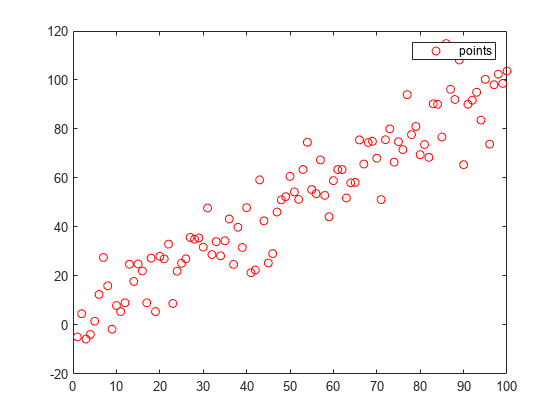• Method1

Find the right singular vector corresponding to the largest singular value, i.e., the principal direction.

points = [x',y'];
A =  points- mean(points);
[U,S,V] = svd(A);
direction = V(:,1);% 主元方向
t = -10:100;
x_ = direction(1).*t;
y_ = direction(2).*t;
plot(x_,y_,'b-',DisplayName="svd fit")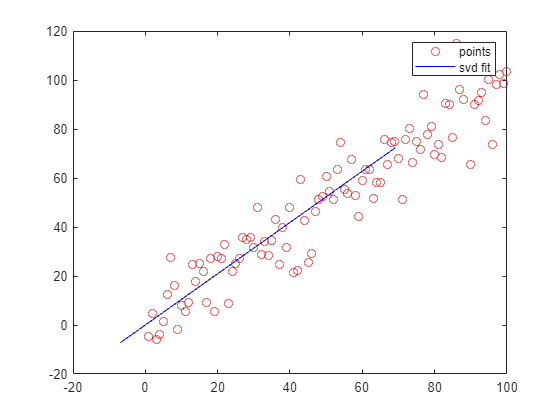legend;
• Method2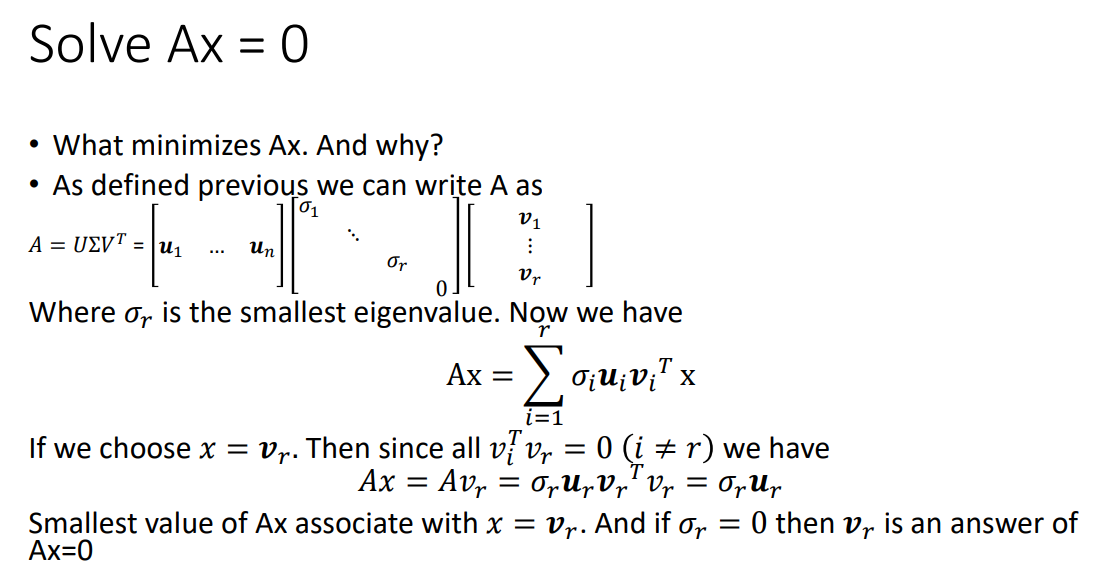<math-renderer class="js-display-math" style="display: block" data-static-url="https://github.githubassets.com/static" data-run-id="17bf8fd32ee913839a0a5e61ff24f77b">$$\left\lbrack U,S,V\right\rbrack =\textrm{svd}\left(A\right)$$</math-renderer>

I tried to make the <math-renderer class="js-inline-math" style="display: inline" data-static-url="https://github.githubassets.com/static" data-run-id="17bf8fd32ee913839a0a5e61ff24f77b">$\textrm{Ax}=0$</math-renderer> form of non-zero least squares solution (the optimal solution is the column vector corresponding to the smallest singular value of V), so it was designed to be solved in the general form $ax+by+c=0$, but because a, b, c variables are not independent unless the constraints are imposed <math-renderer class="js-inline-math" style="display: inline" data-static-url="https://github.githubassets.com/static" data-run-id="17bf8fd32ee913839a0a5e61ff24f77b">$a^2 +b^2 +c^2 =1$</math-renderer>, then it becomes a non-linear least squares, and so it was designed to be ineffective in the general form.

For the above data set and the general formula, if slightly modified, so that c = 1, the general formula becomes <math-renderer class="js-inline-math" style="display: inline" data-static-url="https://github.githubassets.com/static" data-run-id="17bf8fd32ee913839a0a5e61ff24f77b">$a\ast x+b\ast y+1=0$</math-renderer>, this formula can express any plane can not cross the origin of the straight line, but the above data set to fit the straight line is obviously close to the origin, so the general formula is also invalid.

In summary, according to the characteristics of the data set, the general formula is best designed as a form <math-renderer class="js-inline-math" style="display: inline" data-static-url="https://github.githubassets.com/static" data-run-id="17bf8fd32ee913839a0a5e61ff24f77b">$y=k*x+b$</math-renderer>, this formula can express any straight line in the plane can not be perpendicular to the <math-renderer class="js-inline-math" style="display: inline" data-static-url="https://github.githubassets.com/static" data-run-id="17bf8fd32ee913839a0a5e61ff24f77b">$x$</math-renderer> axis. At this point the SVD solves for the non-chiral linear least squares solution <math-renderer class="js-inline-math" style="display: inline" data-static-url="https://github.githubassets.com/static" data-run-id="17bf8fd32ee913839a0a5e61ff24f77b">$\textrm{Ax}=b$</math-renderer>.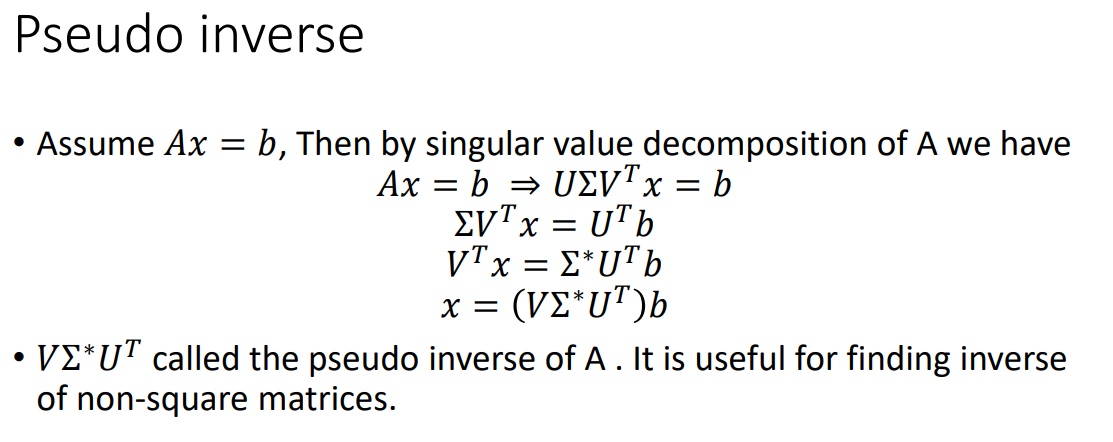% https://ww2.mathworks.cn/matlabcentral/answers/66555-using-svd-to-solve-systems-of-linear-equation-have-to-implement-direct-parameter-calibration-method
x = 1:100;
y = x+10*randn(1,100);
figure;plot(x,y,'ro');hold on;
A = [x',ones(length(x),1)];
b = y';
[U,S,V] = svd(A);
coff = (V*pinv(S)*U')*b; % or use coff = A\b;
fimplicit(@(x,y)coff(1)*x+coff(2)-y,DisplayName="svd ls fit")
legend;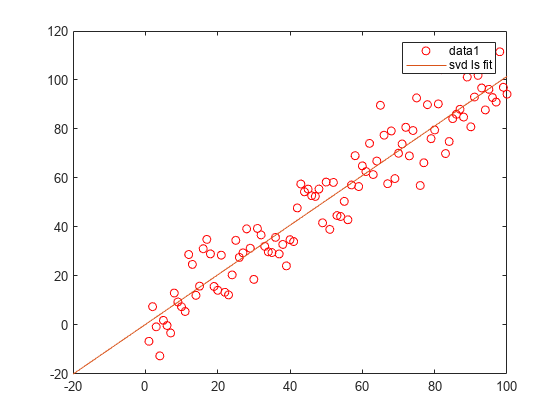## 3D point set linear fitting

%% fit 3d line
x = (1:100)+10*randn(1,100);
y = (1:100)+10*randn(1,100);
z = (1:100)+10*randn(1,100);
figure;
plot3(x,y,z,'b.')
grid on;hold on;

points = [x',y',z'];
avg = mean(points,1);
subtracted = points-avg;
[~,~,V] = svd(subtracted);
direction = V(:,1);
t = -100:100;
x_ = avg(1)+direction(1)*t;
y_ = avg(2)+direction(2)*t;
z_ = avg(3)+direction(3)*t;
plot3(avg(1),avg(2),avg(3),'ro')
plot3(x_,y_,z_,DisplayName="svd fit")
legend;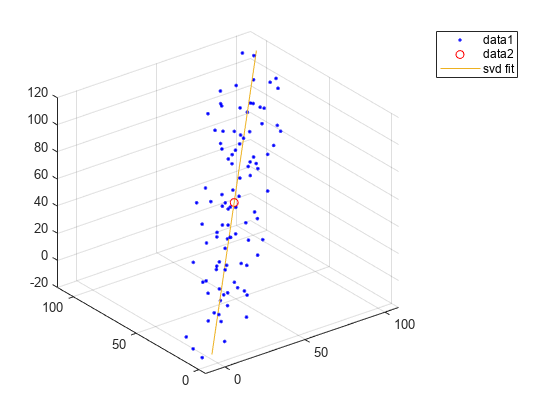## References

http://graphics.ics.uci.edu/ICS6N/NewLectures/Lecture19.pdf

What is benefit to use SVD for solving Ax=b

### 인용 양식

cui,xingxing (2023). SVD-Fit-Line (https://github.com/cuixing158/SVD-Fit-Line/releases/tag/v0.01), GitHub. 검색됨 .

개발 환경: R2023a
모든 릴리스와 호환
##### 플랫폼 호환성
Windows macOS Linux

### Community Treasure Hunt

Find the treasures in MATLAB Central and discover how the community can help you!

Start Hunting!
버전 게시됨 릴리스 정보
0.01

이 GitHub 애드온의 문제를 보거나 보고하려면 GitHub 리포지토리로 가십시오.
이 GitHub 애드온의 문제를 보거나 보고하려면 GitHub 리포지토리로 가십시오.